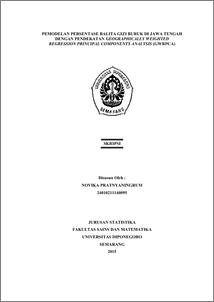# PEMODELAN PERSENTASE BALITA GIZI BURUK DI JAWA TENGAH DENGAN PENDEKATAN GEOGRAPHICALLY WEIGHTED REGRESSION PRINCIPAL COMPONENTS ANALYSIS (GWRPCA)

PRATNYANINGRUM, NOVIKA (2015) PEMODELAN PERSENTASE BALITA GIZI BURUK DI JAWA TENGAH DENGAN PENDEKATAN GEOGRAPHICALLY WEIGHTED REGRESSION PRINCIPAL COMPONENTS ANALYSIS (GWRPCA). Undergraduate thesis, FSM Universitas Diponegoro.Preview
PDF
2521Kb

## Abstract

Geographically Weighted Regression Principal Components Analysis (GWRPCA) is a combination of method of Principal Components Analysis (PCA) and Geographically Weighted Regression (GWR). PCA is used to eliminate the multicollinearity effect in regression analysis. GWR is a local form of regression and a statistical method used to analyze the spatial data. In GWRPCA predictor variables is a principal components of the PCA result. Estimates of parameters of the GWRPCA model can use Weighted Least Square (WLS). Selection of the optimum bandwidth use Cross Validation (CV) method. Conformance testing PCA regression and GWRPCA models approximated by the F distribution, while the partial identification of the model parameters using the t distribution. In PCA obtained variables that affect the percentage of severe children malnutrition in Central Java in 2012 can be represented or replaced with PC1 and PC2 which can explain the total variance of data is 78.43%. Application GWRPCA models at the percentage of severe children malnutrition in Central Java in 2012 showed every regency locations have different model with global coefficient of determination is 0.6313309 and the largest local coefficient of determination is 0.72793026 present in Batang regency, while the smallest local coefficient of determination is 0.03519539 present in Sukoharjo regency. Keywords : Severe Malnutrition, Multicollinearity, Geographically Weighted Regression Principal Components Analysis, Weighted Least Square, Coefficient of Determination.

Item Type: Thesis (Undergraduate) H Social Sciences > HA Statistics Faculty of Science and Mathematics > Department of Statistics 47169 Mr Hasbi Yasin 22 Dec 2015 15:16 22 Dec 2015 15:16

Repository Staff Only: item control page